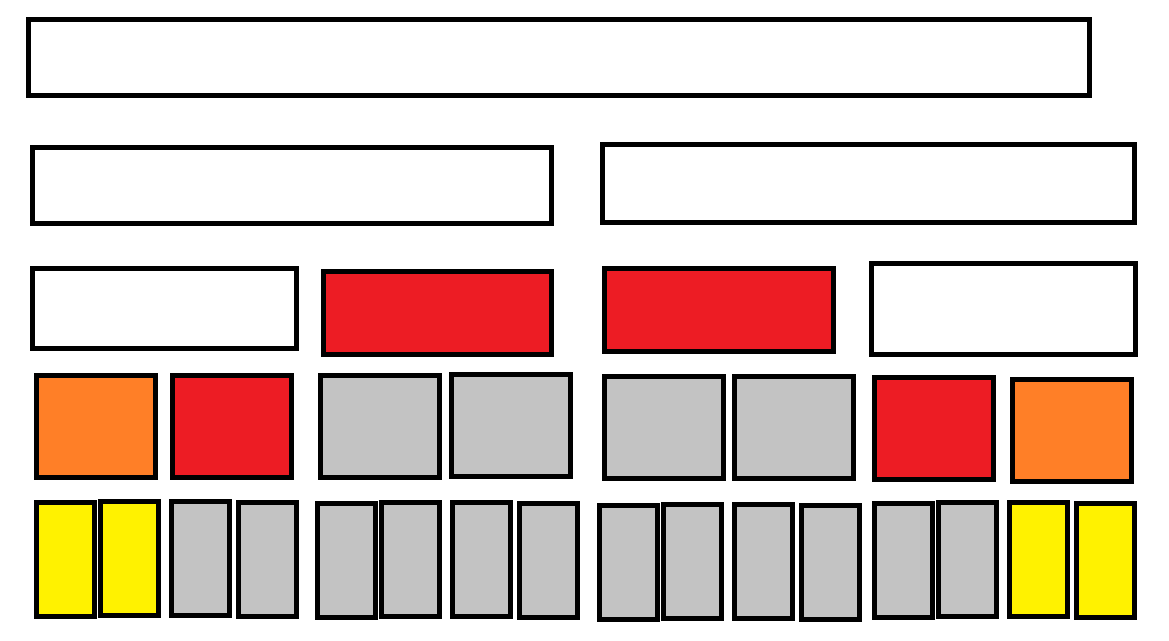# x义x的博客

……就算是单程票，也是可以的吧？

0%

T1代码 T1题解 T2代码 T2题解 T3代码 T3题解
ZJOI2019 Day1 $\sqrt{}$ $\sqrt{}$ $\sqrt{}$ $\sqrt{}$ $\sqrt{}$ $\sqrt{}$
ZJOI2019 Day2 $\sqrt{}$ $\sqrt{}$ $\sqrt{}$ $\sqrt{}$

# ZJOI2019 Day1

## T2• 白色
• 红色
• 灰色
• 黄色
• 橙色

## T3

“能”字在这里可能引发误解：对于每个集合，策略是完全确定的，不会有哪个集合既“能”使 $v\le W$ 又“能”使 $v>W$。